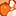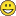# QlikView App Dev

Discussion Board for collaboration related to QlikView App Development.

Announcements
cancel
Showing results for
Did you mean:Creator

## how to loop through multiple csv files

Hi everyone.

So I have the following files:Now what I want to do is to loop through each of them.

The thing is that there is no date column inside each csv. So I cant just link these csv's on date.

what I would like to do is to store each csv's date part (vch_soh_20150803 , vch_soh_20150804 , etc) in a variable whilst looping through all of them whilst concatenating them all to make one table.

I would then use the the variable that holds the date to filter on each csv.

I'm assuming as soon as I store one file's date as a variable, it will overwrite the previous file's date.

What would be the best way of doing this? would it be better to rather add the date as a column on the database side?

Thanks,

Stefan

1 Solution

Accepted SolutionsPartner

Stefan

I assume you mean that you want to store the file date in a field. Something like this:

For Each vFile in FileList('<path to your files>\*.csv')

Let vBasename = SubField(vFile, '\', -1)   // get base file name

Let vDate = Num(Date#(Mid(vBasename, 9, 8), 'YYYYMMDD')));   // get date part as numeric

Data:

LOAD \$(vDate) As Filedate,      // store as field

*

FROM [\$(vFile)]

(txt,  ...);                                   //complete the ... with the attributes you get from the load wizard

Next

Logic will get you from a to b. Imagination will take you everywhere. - A Einstein
4 RepliesMVP

You can loop over all files using ForEach  vFile in FileList(..) ... Next vFile

(There is an example in the HELP for the ForEach statement and FileList function.)

Inside the ForEach loop, concatenate all csv files and add a record to a QV table with the Date part of the filename (wich you can retrieve using subfield() function).

Then afterward to what you want.Master III

set i=0;

set vConcatenate='';

sub GetFileNames(DirName,extension)

for each FoundFile in filelist(DirName & extension)

if i>0 then

let vConcatenate='Concatenate';

End if

let i=i+1;

let vFileName=subfield(TextBetween('\$(FoundFile)','\','.'),'\',-1);

LET vStartTime = Now();

\$(Include='\$(vFilePath)');

LET vEndTime = Now();

Sales:

'\$(vStartTime)' AS StartTime,

'\$(vEndTime)' AS EndTime

AutoGenerate (1);

next FoundFile

end sub

Call GetFileNames('C:\Data\','*.csv');Partner

Stefan

I assume you mean that you want to store the file date in a field. Something like this:

For Each vFile in FileList('<path to your files>\*.csv')

Let vBasename = SubField(vFile, '\', -1)   // get base file name

Let vDate = Num(Date#(Mid(vBasename, 9, 8), 'YYYYMMDD')));   // get date part as numeric

Data:

LOAD \$(vDate) As Filedate,      // store as field

*

FROM [\$(vFile)]

(txt,  ...);                                   //complete the ... with the attributes you get from the load wizard

Next

Logic will get you from a to b. Imagination will take you everywhere. - A EinsteinCreator
Author

Hi thank you so much Jonathan! This is exactly what I wanted to doIt worked perfectly.

kind regards,

Stefan